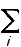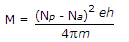# Materials and Components – Section 3(Page 4)

Materials and Components – Section 3

31. Thermistor is used in
 A. temperature measurement B. temperature control C. high temperature alarm circuits of devices D. all of the above

32. Nichrome is used for
 A. overhead line wires B. heater coils C. lamp filaments D. all of the above

33. The electric dipole moment of a neutral system of point charges Q1, Q2…. isQiri . The charges Qi
 A. must be all positive B. must be all negative C. must be such thatQi = 0 D. either (a) or (b)

Explanation:

The system is neutral and hence sum is zero.

34. The class of insulation of impregnated paper is
 A. A B. B C. C D. F

35.Assertion (A): The resistance of a copper specimen changes by about 22% when temperature is increased from room temperature to 700°C.

Reason (R): The physical properties of a material are determined mostly by the extent of impurities.

 A. Both A and R are true and R is correct explanation of A B. Both A and R are true but R is not correct explanation of A C. A is true but R is false D. A is false but R is true

Explanation:

The increase of resistance with temperature is governed by temperature coefficient of resistance.

36. Assertion (A): For most metals, Fermi energy EF is less than 10 eV.

Reason (R): Fermi level of a metal is given by EF = 3.64 x 10-19 (n)2/3 where n is number of free electrons/m3 of metal.

 A. Both A and R are true and R is correct explanation of A B. Both A and R are true but R is not correct explanation of A C. A is true but R is false D. A is false but R is true

37. The capacitance of an insulated conducting sphere of radius R in vacuum is
 A. 2p∈0R B. 4p∈0R C. 4p∈0R2 D. 4p38. The transition of electron from energy level W1 to W2 is associated with emission or absorption of electro magnetic radiation of frequency f such that
 A. hf = |W1 – W2| B. |W1 – W2|f = h C. hf = 0.5|W1 – W2| D. (hf)2 = |W1 – W2|

39. A material has N spins per m3. If Np are the dipoles per m3 parallel to field and Na are the dipoles per m3 anti-parallel to field, the magnetisation is
 A.B.C.D.